## Plus Blog

December 8, 2013

An infinite set is called countable if you can count it. In other words, it's called countable if you can put its members into one-to-one correspondence with the natural numbers 1, 2, 3, ... . For example, a bag with infinitely many apples would be a countable infinity because (given an infinite amount of time) you can label the apples 1, 2, 3, ... and so on.

Two countably infinite sets A and B are considered to have the same "size" (or cardinality) because you can pair each element in A with one and only one element in B so that no elements in either set are left over. This idea seems to make sense, but it has some funny consequences. For example, the even numbers are a countable infinity because you can link the number 2 to the number 1, the number 4 to 2, the number 6 to 3 and so on. So if you consider the totality of even numbers (not just a finite collection) then there are just as many of them as natural numbers, even though intuitively you'd think there should only be half as many.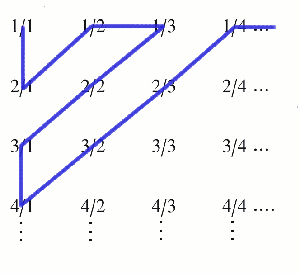Something similar goes for the rational numbers (all the numbers you can write as fractions). You can list them as follows: first write down all the fractions whose denominator and numerator add up to 2, then list all the ones where the sum comes to 3, then 4, etc. This is an unfailing recipe to list all the rationals, and once they are listed you can label them by the natural numbers 1, 2, 3, ... . So there are just as many rationals as natural numbers, which again seems a bit odd because you'd think that there should be a lot more of them.

It was Galileo who first noticed these funny results and they put him off thinking about infinity. Later on the mathematician Georg Cantor revisited the idea. In fact, Cantor came up with a whole hierarchy of infinities, one "bigger" than the other, of which the countable infinity is the smallest. His ideas were controversial at first, but have now become an accepted part of pure mathematics.

You can find out more about all this in our collection of articles on infinity.

December 7, 2013We've all got favourite numbers and some of us even go as far as thinking some numbers lucky or unlucky. Seven is probably one that crops up quite a lot in this context, so it's nice to know that it really is a lucky number. No, seriously, that's true! There is a mathematical recipe for finding lucky numbers, and 7 is one of them.

The recipe goes like this. Write down all the positive whole numbers starting from 1. First, you eliminate all even numbers, leaving only the odds:

1, 3, 5, 7, 9, 11, 13, 15, ... .

The next number on the list after 1 is 3, so you now go and eliminate every third number, leaving:

1, 3, 7, 9, 13, 15, ... .

The next surviving number after 3 is 7, so you eliminate every 7th number in the list of survivors. And so on. When you've finished this process the sequence of surviving numbers starts:

1, 3, 7, 9, 13, 15, 21, 25, 31, 33, 37, 43, 49, 51, 63, 67, 69, 73, 75, 79, 87, 93, 99, ... .

These surviving numbers are called lucky numbers. They are similar to the prime numbers in some ways. There are infinitely many of them, and they are the result of a sieving process (successively eliminating numbers) similar to the sieve of Eratosthenes which sieves out primes. When you try and approximate the distribution of the lucky numbers among the other numbers as you move up the number line, the result is akin to the prime number theorem. The lucky numbers even have their own version of the Goldbach conjecture, which states that any odd number greater than 4 can be written as the sum of two primes. One thing that isn't known, though, if whether there are infinitely lucky numbers that are also prime, or only finitely many.

All this doesn't make 7 lucky in the everyday sense of the word — but it makes us feel very lucky to have so many numbers to play with!

December 6, 2013

Now here's an important mathematical question: how likely are you to win the lottery?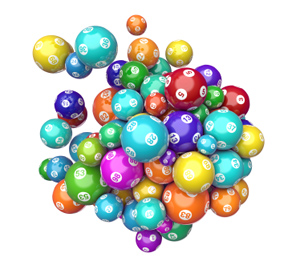1 in 14 million.

In the UK lottery you have to choose 6 numbers out of 49, and for a chance at the jackpot you need all of your 6 numbers to come up in the main draw. So the question is really how many possible combinations of 6 numbers can be drawn out of 49? There are 49 possibilities for the first number, 48 for the second, and so on, to 44 possibilities for the sixth number, so there are 49x48x47x46x45x44=10,068,347,520 ways of choosing those six numbers... in that order.

But we don't care which order our numbers are picked, and the number of different ways of ordering 6 numbers are 6x5x4x3x2x1=6! =720: there are six possibilities for the number that comes first in the list, 5 for the number that comes second, and so on. Therefore our six numbers are one of 49x48x47x46x45x44/6! = 13,983,816 so we have about a one in 14 million chance of hitting the jackpot. Hmmm...

But on a brighter note, we have just discovered a very useful mathematical fact: the number of combinations of size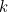(sets of objects in which order doesn’t matter) from a larger set of size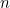is: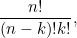where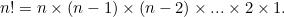This sort of argument lies at the heart of combinatorics, the mathematics of counting. It might not help you win the lottery, but it might keep you healthy. It is used to understand how viruses such as influenza reproduce and mutate, by assessing the chances of creating viable viruses from random recombination of genetic segments.

You can read more on the uses of combinatorics, including in money matters (lottery), love (well, kissing frogs) and fun (juggling and Rubik's cubes):

Combinatorics on Plus

December 5, 2013

Triangles do it, squares do it, even hexagons do it — but pentagons don't. They just won't fit together to tile your bathroom wall. That's the reason why you'll find it very difficult to find pentagonal tiles in any hardware shop.

It's actually really easy to see why pentagons won't tile the plane. We are talking regular pentagons here, shapes that have five sides of equal length and angles between them. In a regular pentagon the internal angle between two sides is 108°. In a regular tiling, adjacent tiles share whole edges, rather than just parts of edges, so corners of tiles meet corners of other tiles. To fit a number of tiles around a corner point, their internal angles must add up to 360°, since that's a full turn. If you try to fit three pentagons, you only get 3 x 108° = 324°, so there is a gap. If you fit four pentagons, you get 4 x 108° = 432°, so two of them overlap. The same isn't true for equilateral triangles, squares, or regular hexagons. Here the internal angles are 60°, 90° and 120° respectively, so you can fit six triangles, four squares and three hexagons around a corner point. (You can try and work out for yourself if any other regular polygons can give you a regular tiling.)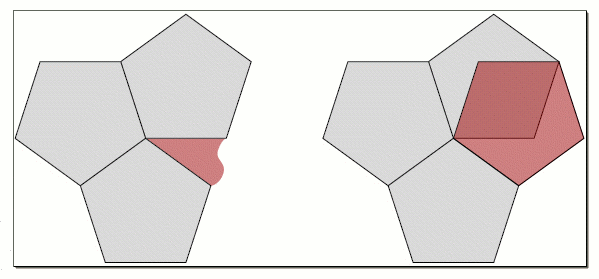Three pentagons arranged around a point leave a gap, and four overlap. Image: Craig Kaplan.

If pentagons don't work can we perhaps use other shapes with five-fold symmetry to tile the plane? Find out more in The trouble with five.

December 4, 2013

Remember maps? In the old days, before smart phones and satnavs, we used to use them to get around. They posed quite a few problems, including folding them and understanding them. To help with the latter, map makers often use different colours to colour adjacent regions, be they countries, counties, or parts of a city. And pretty much since the beginning of map making, they have known that they will never need more than four colours to colour a whole map. It's something that's so blindingly obvious once you start playing with a few maps, it should be easy to prove mathematically: when colouring a map drawn on a flat piece of paper, four colours will always suffice.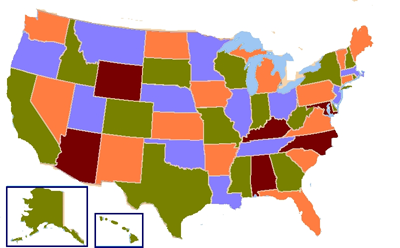The US in four colours. Image: Wikipedia.

Mathematicians first set themselves the task back in 1852, and it proved a massive headache. It took 24 years for the first proof to be announced, only to be found faulty another eleven years later. Then it took another 86 years, until 1976, until the mathematicians Kenneth Appel and Wolfgang Haken came up with a second proof. They first supposed that there are maps that need five colours, from which you can then choose one that has the smallest number of countries. They then showed that such a minimal map must contain one of 1,936 possible configurations; and they also proved that every one of these possible configurations can be reduced into a smaller configuration which also needs five colours. This is a contradiction because they assumed that they had already started with the smallest five-colour map. When you find a contradiction you can deduce that your initial assumption, that there are maps that need five colours, is false.

This sounds good, but there was a hitch. The part of their proof which showed that these 1,936 configurations could be reduced was done by a computer, which simply ploughed through every configuration and checked it. No human being could in their lifetime ever actually read the entire proof to check that it was correct. This caused an outcry: if nobody can check it, how can we ever know it doesn't contain a mistake? Can mathematics ever be done by computers?

The proof has since been improved and verified independently (by computers), so most people are quite happy to consider the result proven. The question of whether computer proofs can ever be considered sound, however, is still a hot one.

To find out more, read The philosophy of proof and Welcome to the maths lab.

December 3, 2013

Here's a little game to play. Take the interval from 0 to 1, including the end points. Now delete the middle third of this interval, from 1/3 to 2/3, but without deleting the two end points 1/3 and 2/3. Then delete the middle thirds of the two intervals that are left, [0,1/3] and [2/3,1], again without deleting the end points. Keep going, deleting middle thirds ad infinitum — well, you can't really do this in practice, but you can imagine it. What will you be left with in the end?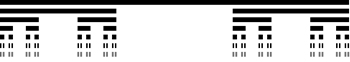Seven steps towards C.

Let's call the set of left-over points C. Clearly, C contains the end points of middle third intervals because these don't get removed: 0, 1, 1/3, 2/3, 1,9, 2/9, 7/9, 8/9, and so on. But other points are left too. For example, 1/4 does not get removed at the first step because it's less than 1/3, it does not get removed at the second step either because it's bigger than 2/9, and so on.

Does C contain any intervals at all? It's pretty easy to convince yourself that it doesn't: any line segment, no matter how tiny, will have been broken up by having its middle third removed. C is what's called totally disconnected because all its connected components are just points.

But these points are not isolated. If you draw a little circle around any point x in C, then no matter how small the circle, it will contain other points of C. For example, take the point 0. Arbitrarily close to it there is a point of the form 1/3k for some k, which is in C because it's the end point of a middle third interval. The fact that every point has other points arbitrarily close to it makes C into a perfect set.

All this means that C inhabits a strange world between dimensions. It's more than a collection of isolated points, which has dimension 0, and less than a line, which has dimension 1. If you calculate its Hausdorff dimension, which is an extension of our usual concept of dimension, the answer is ln(2)/ln(3)=0.63, which is between 0 and 1 as expected.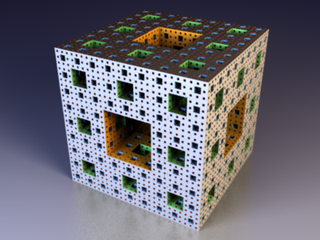The Menger sponge. Image: Niabot.

The set C is also self-similar. For example, if you take the part of C contained in some tiny interval [0,1/3k] and blow it up, what you get looks exactly the same as C itself. Being self-similar and having a dimension that's not a whole number makes C into a fractal.

C has a name too: it's called the middle-thirds Cantor set, after Georg Cantor, who wrote about it in 1883, although it had previously been discovered by Henry John Stephen Smith. Cantor was one of the first people to give a rigorous description of a fractal, though the term "fractal" was not coined until almost 100 years later by Benoit Mandelbrot.

Cantor sets often turn up in mathematics when things are being broken up according to the same recipe ad infinitum. For example, you can create a similar construct in two dimensions by dividing a square up into nine smaller squares and repeatedly removing the middle one. The result is called the Sierpinski carpet, which has a Hausdorff dimension of ln(8)/ln(3)=1.892. The three-dimensional analogue is the Menger sponge.